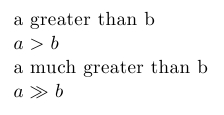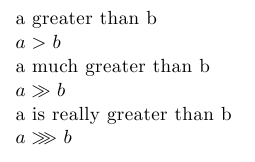greater than sign(s)

# LaTeX greater than signs

StartLaTeX faqLaTeX topicsLaTeX course

< less than     > logical and, or, not

## greater than

If a is greater than b you could wrote a > b just use the normal char > ; if a is much greater than b then a » b where « would be the command \gg; and finally if a is really great compared with b then you could use the \ggg command which requires usepackage amssymb.

### example

\documentclass{article}
\begin{document}
\ \\
a greater than b \\
$a > b$ \\
a much greater than b \\
$a \gg b$ \\
\end{document}

Output:### example really greater than

\documentclass{article}
\usepackage{amssymb}
\begin{document}
\ \\
a greater than b \\
$a > b$ \\
a much greater than b \\
$a \gg b$ \\
a is really greater than b \\
$a \ggg b$\\
\end{document}

Output: